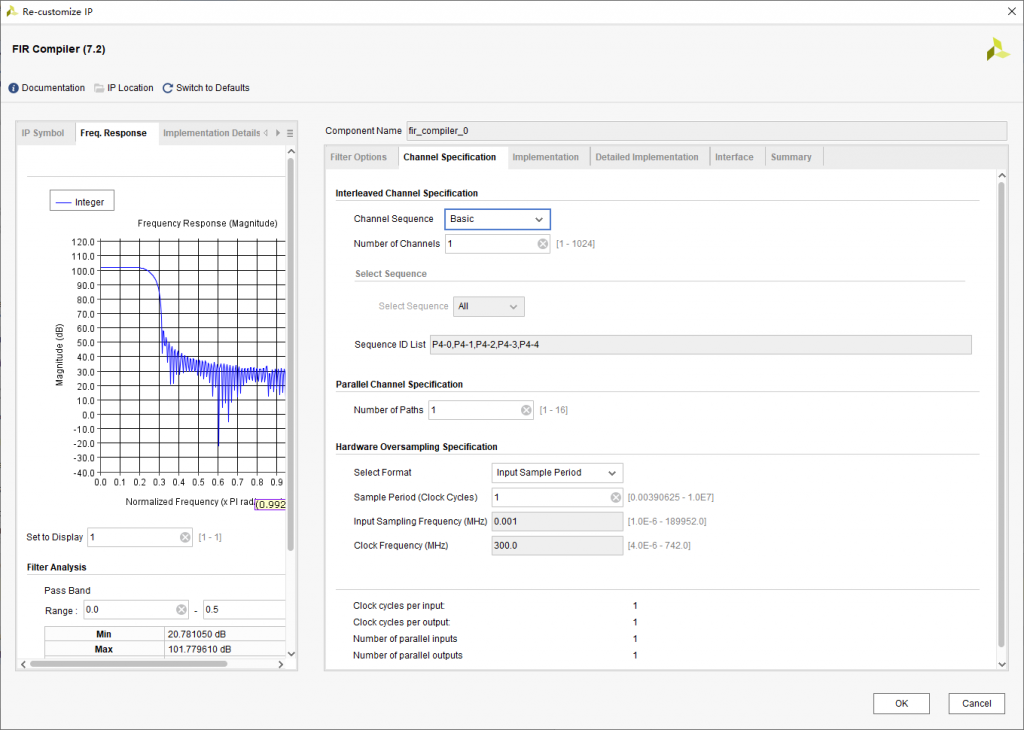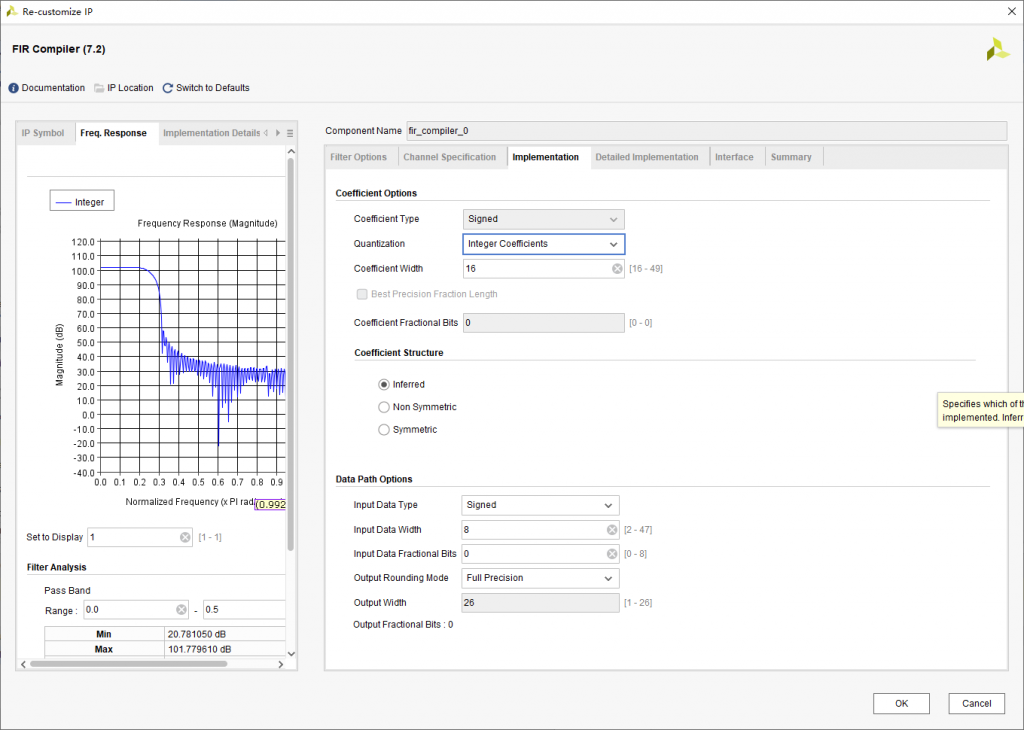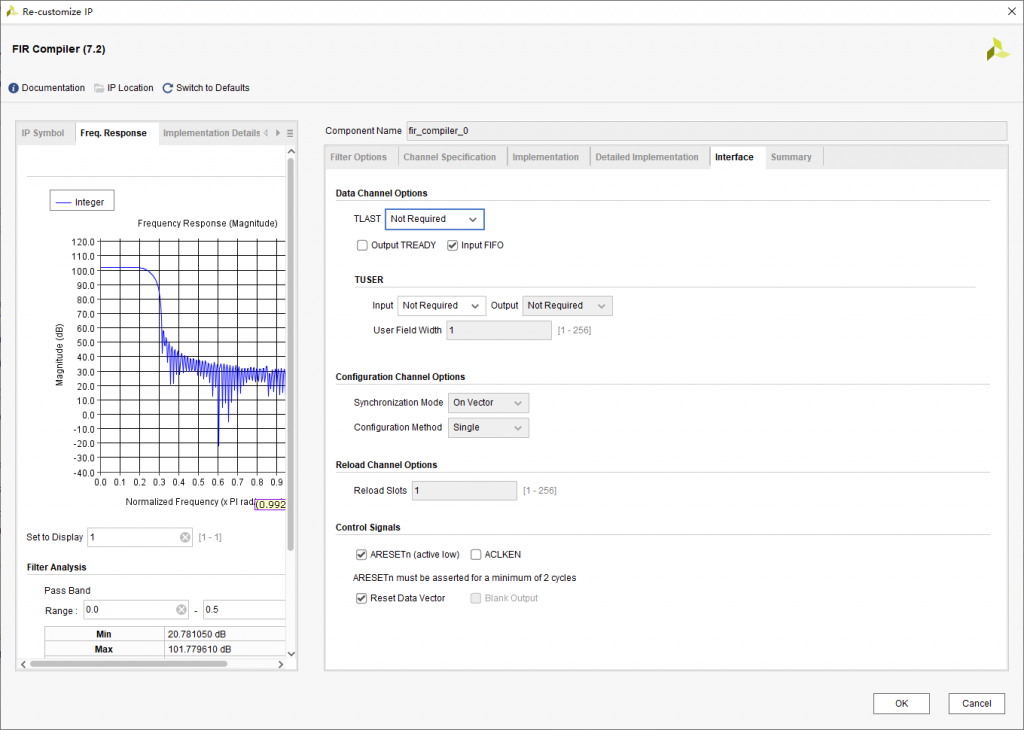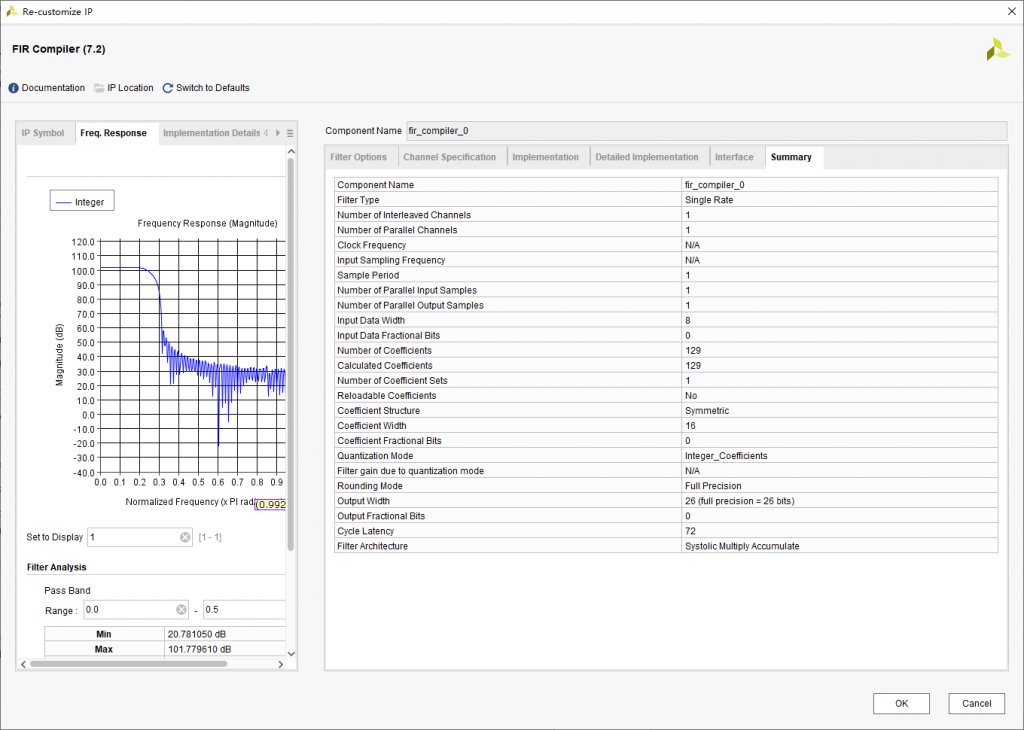# 成形滤波器的研究与设计

### 1. 概述

（1）频谱压缩，限制信号带宽。在数字通信中基带信号是矩形脉冲，突变的上升沿和下降沿包含高频分量丰富，其频谱范围普遍比较宽（频谱是一个Sa函数）。为了有效利用信道，在信号传输之前，需要对信号进行频谱压缩。使其在消除码间串扰和达到最佳检测的前提下，大大提高频带利用率。信号带宽匹配信道带宽。

（2）改变传输信号的成形波形，可以减小抽样定时脉冲误差所带来的影响，即降低了码间干扰（ISI）。信号带限就会引入码间串扰（时域的离散化对应频域的周期化），会导致接收信号波形失真。但一般情况下，只需要在特定时刻的信号抽样值无失真，并不需要整个信号波形都无失真，而升余弦滤波器刚好就能对基带信号频谱进行带限，并且不影响信号在特定时刻的抽值。

### 2. 成形滤波器的原理

y(0)=a0*x(0) =-2529

y(1)=a0*x(1)+a1*x(0)=2529

y(2)=a0*x(2)+a1*x(1)+a2*x(0)=7183

y(3)=a0*x(3)+a1*x(2)+a2*x(1)+a3*x(0)=8054

y(4)=……=2357

y(5)=……=-10581

### 3. 成形滤波器在FPGA上的实现

(1) 首先生成20000个1 -1的随机序列，并保存在rand_data.txt文件中，Matlab代码如下：

```clear;clc;
N=20000;
s=randi([0 1],N,1);
s1=2*s-1;
fid=fopen('D:\Temp\matlab\rand_data.txt','w');
fprintf(fid,'%d\r\n',s1);fclose(fid);```

```clear;clc;
span=32; %符号跨度
sps=4; %每个符号的点采样数
%使用rcosdesign得到滤波器系数
h=rcosdesign(0.25, span, sps, 'sqrt');
%得到的系数通带增益为6dB，暂不清楚原因，除2后正常
h2=h/2;
figure (1);
freqz(h2,1,1024);
%将系数放大并取整
coe_int=round((h/max(abs(h)))*(2^15-1));
freqz(coe_int,1,1024);
format long;
%将系数量化为15位小数
coe_frac=coe_int/2^15;
figure (2);
freqz(coe_frac,1,1024);
fid=fopen('D:\Temp\matlab\coe_frac.coe','w');
fprintf(fid,'CoefData =\r\n');
fprintf(fid,'%16.15f,\r\n',coe_frac);fprintf(fid,';');fclose(fid);
fid=fopen('D:\Temp\matlab\coe_int.coe','w');
fprintf(fid,'CoefData =\r\n');
fprintf(fid,'%d,\r\n',coe_int);fprintf(fid,';');fclose(fid);
fid=fopen('D:\Temp\matlab\coe_int.txt','w');
fprintf(fid,'%d ',coe_int);fclose(fid);```(4) 编写testbench，读入第(1)步生成的rand_data.txt，并将FIR滤波后的结果保存在filt_data.txt中，部分代码如下

```integer fid_in;
initial
begin
fid_in = \$fopen("D:/Temp/matlab/rand_data.txt","r");
end

always@(posedge clk_1m)
begin
if(!rst)
begin
din <= 8'd0;
s_data_tvalid <= 1'b0;
end
begin
\$fscanf(fid_in,"%d",din);
s_data_tvalid <= 1'b1;
end
end

integer fid_out;
initial
begin
fid_out = \$fopen("D:/Temp/matlab/filt_data.txt","w");
end
always@(posedge clk_4m)
begin
if(m_data_tvalid)
begin
\$fwrite(fid_out,"%d\n",dout);
end
end```

(6) 使用对比filt_data.txt与Matlab使用filter函数得到的结果是否一致，代码如下

```clear;clc;
ps=1*10^6; %码速率为1MHz
Fs=4*10^6; %采样速率为8MHz
N=2000; %仿真数据的长度
coe_int=importdata('D:\Temp\matlab\coe_int.txt');
s=importdata('D:\Temp\matlab\rand_data.txt');
fir_out=importdata('D:\Temp\matlab\filt_data.txt');
t=0:1/Fs:(N*Fs/ps-1)/Fs; %产生长度为N,频率为fs的时间序列
%截断FIR输出的前8K数据
fir_out_8k_temp=fir_out(2:N*(Fs/ps)+1,1);
fir_out_8k=fir_out_8k_temp';
%以Fs频率采样
ups=upsample(s',Fs/ps);
%滤波
filt_mat=filter(coe_int,1,ups);
filt_mat_8k=filt_mat(1:8000);
%对比数据
isequal(fir_out_8k,filt_mat_8k)```